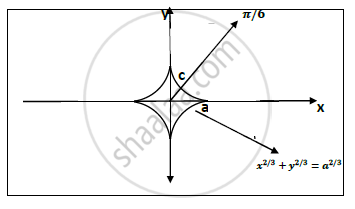# Prove that for an Astroid X 2 3 + Y 2 3 = a 2 3 the Line 𝜽=𝝅/𝟔 Divide the Arc in the First Quadrant in a Ratio 1:3. - Applied Mathematics 2

Prove that for an astroid     x^(2/3) +y2/3= a^(2/3) the line 𝜽=𝝅/𝟔 Divide the arc in the first quadrant in a ratio 1:3.

#### Solution

Given curve : astroid x^(2/3)+y^(2/3) = a^(2/3)

The line 𝜽=𝝅/𝟔 cuts the asroid in 1 st quadrant.C is the point on the curve which cuts the arc.
Length of astroid in first quadrant:

Put   x = acos^3t and y=asin^3t

dx=-3 asin t.cos^2tdt    dy=3 acos t.sin^2tdt

s= int_0^(pi/2) sqrt ((dx/dt)^2+(dy/dt)^2)=int_0^(pi/2) sqrt((-3asin t.cos^2 t)^2+(3 acos t .sin^2 t )^2) dt

= int_0^(pi/2) 3a.sin t.cost dt

= 3/2 a  int_0^(pi/2) sin 2t  dt

=3/4 a [-cos 2t ]_0^(pi/2)

∴ s= 3/2 a                         ………………….(1)

Now the length of the curve ac : Just put pi/6 𝒊𝒏𝒔𝒕𝒆𝒅 𝒐𝒇 pi/2  because the curve is Only upto given line.

∴ S(ac) =int_0^(pi/6) 3a sint . cost dt =3/4a [-cos 2t]_0^(pi/6)

= 3/4 a [-1/2+1]

s(ac)= 3/8 a                                ……………(2)

Legnth of remaining part = 3/2a-3/8 a=9/8 a  ……………….(3)

Divide eqn (3) and (2).
The line pi/6 cuts the given astroid in the ratio of 1:3
Hence proved.

Concept: Exact Differential Equations
Is there an error in this question or solution?